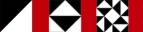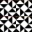Next Higher-Order Parsing 22

# パーサParsers

• この関数を使うと、atomの完全な定義はこうなる

With this definition, a complete definition of atom is:

```        \$atom = alt(lookfor("NUMBER"),
lookfor("VAR"),
conc(lookfor("FUNC"),
lookfor("("),
\$EXPRESSION,
lookfor(")"),
));
```
• \$factorもほとんど同じ

Similarly, here's \$factor:

```     #  factor → atom ("^" NUMBER | nothing)

\$factor = conc(\$ATOM, alt(conc(lookfor("^"),
lookfor("NUMBER")),
\￢hing));
```
• \$termはこう

Here's \$term:

```     #  term → factor ("*" term | nothing)

\$term = conc(\$FACTOR, alt(conc(lookfor("*"), \$TERM),
\&nothing));
```

 NextCopyright © 2007 M. J. Dominus